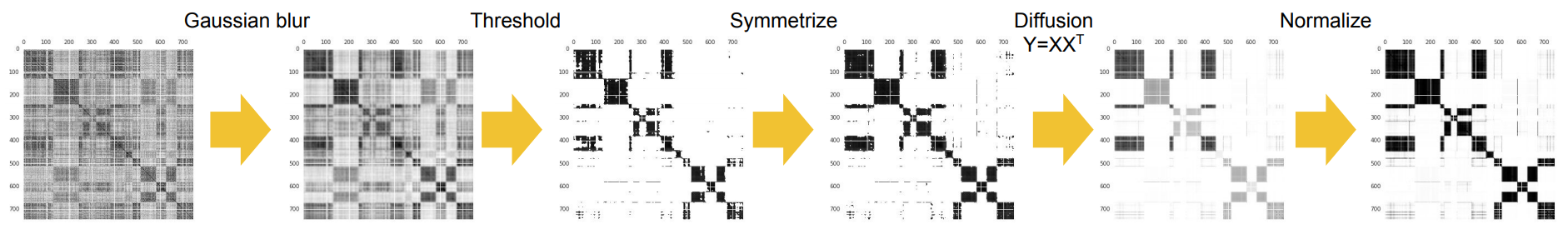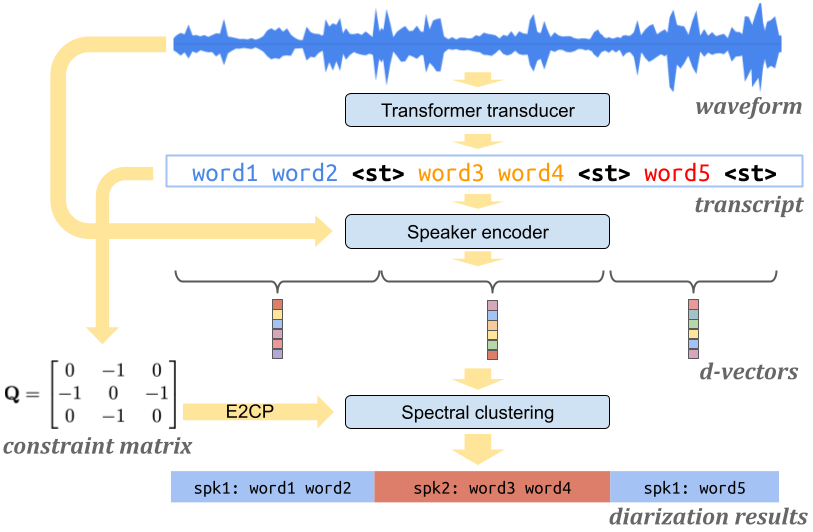Showing:

0

253

10hrs ago

3

0

# Spectral Clustering

## Overview

This is a Python re-implementation of the spectral clustering and constrained spectral clustering algorithms in these two papers:## Notice

We recently added new functionalities to this library to include algorithms in a new paper. We updated the APIs as well.

If you depend on our old API, please use an older version of this library:

``````pip3 install spectralcluster==0.1.0
``````

## Disclaimer

This is not a Google product.

This is not the original C++ implementation used by the paper.

• numpy
• scipy
• scikit-learn

## Installation

Install the package by:

``````pip3 install spectralcluster
``````

or

``````python3 -m pip install spectralcluster
``````

## Tutorial

Simply use the `predict()` method of class `SpectralClusterer` to perform spectral clustering. The example below should be closest to the original C++ implemention used our ICASSP 2018 paper.

``````from spectralcluster import configs

labels = configs.icassp2018_clusterer.predict(X)
``````

The input `X` is a numpy array of shape `(n_samples, n_features)`, and the returned `labels` is a numpy array of shape `(n_samples,)`.

You can also create your own clusterer like this:

``````from spectralcluster import SpectralClusterer

clusterer = SpectralClusterer(
min_clusters=2,
max_clusters=7,
autotune=None,
laplacian_type=None,
refinement_options=None,
custom_dist="cosine")

labels = clusterer.predict(X)
``````

For the complete list of parameters of `SpectralClusterer`, see `spectralcluster/spectral_clusterer.py`.### Refinement operations

In our ICASSP 2018 paper, we apply a sequence of refinment operations on the affinity matrix, which is critical to the performance on the speaker diarization results.

You can specify your refinment operations like this:

``````from spectralcluster import RefinementOptions
from spectralcluster import ThresholdType
from spectralcluster import ICASSP2018_REFINEMENT_SEQUENCE

refinement_options = RefinementOptions(
gaussian_blur_sigma=1,
p_percentile=0.95,
thresholding_soft_multiplier=0.01,
thresholding_type=ThresholdType.RowMax,
refinement_sequence=ICASSP2018_REFINEMENT_SEQUENCE)
``````

Then you can pass the `refinement_options` as an argument when initializing your `SpectralClusterer` object.

For the complete list of `RefinementOptions`, see `spectralcluster/refinement.py`.

### Laplacian matrix

In our ICASSP 2018 paper, we apply a refinement operation `CropDiagonal` on the affinity matrix, which replaces each diagonal element of the affinity matrix by the max non-diagonal value of the row. After this operation, the matrix has similar properties to a standard Laplacian matrix, and it is also less sensitive (thus more robust) to the Gaussian blur operation than a standard Laplacian matrix.

In the new version of this library, we support different types of Laplacian matrix now, including:

• None Laplacian (affinity matrix): `W`
• Unnormalized Laplacian: `L = D - W`
• Graph cut Laplacian: `L' = D^{-1/2} * L * D^{-1/2}`
• Random walk Laplacian: `L' = D^{-1} * L`

You can specify the Laplacian matrix type with the `laplacian_type` argument of the `SpectralClusterer` class.

Note: Refinement operations are applied to the affinity matrix before computing the Laplacian matrix.

### Distance for K-Means

In our ICASSP 2018 paper, the K-Means is based on Cosine distance.

You can set `custom_dist="cosine"` when initializing your `SpectralClusterer` object.

You can also use other distances supported by scipy.spatial.distance, such as `"euclidean"` or `"mahalanobis"`.

### Affinity matrix

In our ICASSP 2018 paper, the affinity between two embeddings is defined as `(cos(x,y)+1)/2`.

You can also use other affinity functions by setting `affinity_function` when initializing your `SpectralClusterer` object.

### Auto-tune

We also support auto-tuning the `p_percentile` parameter of the `RowWiseThreshold` refinement operation, which was original proposed in this paper.

You can enable this by passing in an `AutoTune` object to the `autotune` argument when initializing your `SpectralClusterer` object.

Example:

``````from spectralcluster import AutoTune

autotune = AutoTune(
p_percentile_min=0.60,
p_percentile_max=0.95,
init_search_step=0.01,
search_level=3)
``````

For the complete list of parameters of `AutoTune`, see `spectralcluster/autotune.py`.

### Constrained spectral clusteringIn the Turn-to-Diarize paper, the spectral clustering is constrained by speaker turns. We implemented two constrained spectral clustering methods:

• Affinity integration.
• Constraint propagation (see paper  and ).

If you pass in a `ConstraintOptions` object when initializing your `SpectralClusterer` object, you can call the `predict` function with a `constraint_matrix`.

Example usage:

``````from spectralcluster import constraint

ConstraintName = constraint.ConstraintName

constraint_options = constraint.ConstraintOptions(
constraint_name=ConstraintName.ConstraintPropagation,
apply_before_refinement=True,
constraint_propagation_alpha=0.6)

clusterer = spectral_clusterer.SpectralClusterer(
max_clusters=2,
refinement_options=refinement_options,
constraint_options=constraint_options,
laplacian_type=LaplacianType.GraphCut,
row_wise_renorm=True)

labels = clusterer.predict(matrix, constraint_matrix)
``````

The constraint matrix can be constructed from a `speaker_turn_scores` list:

``````from spectralcluster import constraint

constraint_matrix = constraint.ConstraintMatrix(
speaker_turn_scores, threshold=1).compute_diagonals()
``````

Our papers are cited as:

``````@inproceedings{wang2018speaker,
title={{Speaker Diarization with LSTM}},
author={Wang, Quan and Downey, Carlton and Wan, Li and Mansfield, Philip Andrew and Moreno, Ignacio Lopz},
booktitle={2018 IEEE International Conference on Acoustics, Speech and Signal Processing (ICASSP)},
pages={5239--5243},
year={2018},
organization={IEEE}
}

@article{xia2021turn,
title={{Turn-to-Diarize: Online Speaker Diarization Constrained by Transformer Transducer Speaker Turn Detection}},
author={Wei Xia and Han Lu and Quan Wang and Anshuman Tripathi and Ignacio Lopez Moreno and Hasim Sak},
journal={arXiv preprint arXiv:2109.11641},
year={2021}
}
``````

## Rate & Review

Great Documentation0
Easy to Use0
Performant0
Highly Customizable0
Bleeding Edge0
Responsive Maintainers0
Poor Documentation0
Hard to Use0
Slow0
Buggy0
Abandoned0
Unwelcoming Community0
100No reviews found
Be the first to rate

## AlternativesNo alternatives found

## TutorialsNo tutorials found